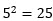# What Is the Exponent Calculator?

The exponent calculator can help you calculate either the base, the power, or, as the name implies, the exponent, based on the two other known variables. It is free, easy to use, and is sure to save you time.

## How to use the exponent calculator?

The concept could not be simpler. All you need to do is enter the two known variables, and the calculator will find the missing one for you.

Exponentiation is a mathematical function that consists of 3 parts: the base, the exponent, and the power. The base is the number that gets multiplied by itself. The exponent is the number that represents how many times the base needs to be multiplied by itself. Finally, the power is the final result or the number you get when you do the multiplication.

So, for example, if you know the base and the power, and you need to find the exponent that makes the statement true, you can easily do so with our calculator. Simply enter the base and the power, and the exponent will be calculated.

Our calculator even works with negative exponents. Basically, when a number has a negative exponent, you need to calculate the reciprocal value of the base:On the other hand, if the base is a negative number, there can be two outcomes. First, if the exponent is an odd number, the result will also be negative, because of the rules of multiplying negative numbers. If, however, the exponent is an even number, the result will be a positive number.

In scientific notation, powers of 10 are used to express either very large (10 with a positive exponent) or very small numbers (10 with a negative exponent).

If the exponent is 0, the result will always be 1. If you are confused as to why this is, you can find the explanation in the full post on our official website.

## What is a fractional exponent?

If the exponent of a number contains a fraction, it is called a fractional exponent. So, what do fractional exponents mean, and how do you calculate with them? To understand how to use them, you need to be familiar with a function similar to exponentiation, which is roots.

Roots are inverse of powers.

So, if:then:So, how does this tie into fractional exponents? Well, we can actually write roots as powers, where the exponent is a fraction. The general formula of this is:To demonstrate this better, we can use the previous example:In most cases, the numerator will be 1. If not, the form is:## How to solve fractional exponents?

Now that we know what fractional exponents are, we can get to solving them. So, what do you do when you see a fractional exponent? The easiest method to solve them is to convert them to roots, as we demonstrated before.

So, for example, if you had 144 ½, you could rewrite it as √144. Now, all you need to do is calculate the square root of 144, which is 12.

Another useful method is for powers that have the same base. Namely, if you’re multiplying two or more powers that have the same base, you can simply copy the base, and add up all the exponents. For adding up fractions, you need to keep in mind that, in order to add them, they need to have the same denominators. If they do not, you need to expand one or more of them, so that they all have the same denominators.

Expanding fractions, sometimes called complicating fractions, is done by multiplying the fraction by another fractions, whose numerator and denominator are equal (the fraction is equal to 1). Since you’re effectively multiplying the fraction by 1, its value doesn’t change, only its form:Let’s do a quick example:The smallest common denominator for 3 and 5 is 15.Now, all we need to do is copy the base and add up the exponents:We can rewrite it as:Scroll to Top